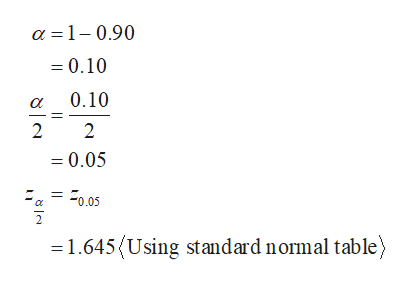# Find the minimum sample size n needed to estimate muμ for the given values of​ c, sigmaσ​, and E.cequals=0.90sigmaσequals=7.3 and Eequals=2Assume that a preliminary sample has at least 30 members.n=?​(Round up to the nearest whole​ number.)

Question
217 views

Find the minimum sample size n needed to estimate
mu
μ for the given values of​ c,
sigma
σ​, and E.

c
equals
=
0.90

sigma
σ
equals
=
7.3
and E
equals
=2
Assume that a preliminary sample has at least 30 members.

n=?

​(Round up to the nearest whole​ number.)

check_circle

Step 1

It is given that confidence level is 0.90, standard deviation is 7.3 and margin of error E is 2.

Step 2

Here,

...help_outlineImage Transcriptionclosea 1-0.90 = 0.10 0.10 2 2 =0.05 a= 0.05 2 1.645 Using standard normal table fullscreen

### Want to see the full answer?

See Solution

#### Want to see this answer and more?

Solutions are written by subject experts who are available 24/7. Questions are typically answered within 1 hour.*

See Solution
*Response times may vary by subject and question.
Tagged in

### Statistics